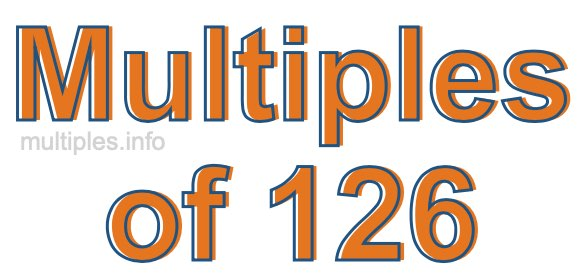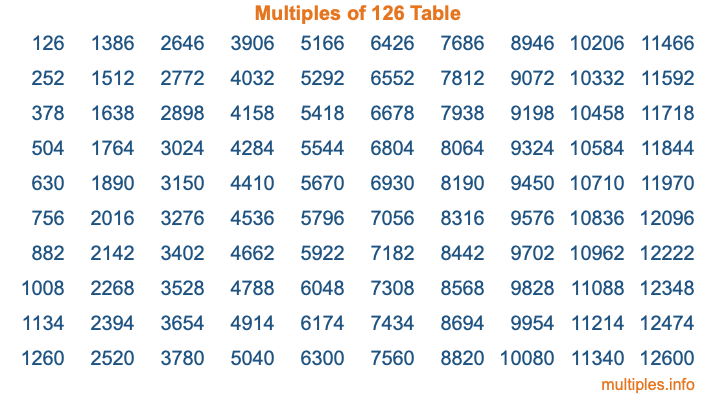Multiples of 126Welcome to the Multiples of 126 page. Here we will first teach you everything you will ever need to know about the multiples of 126, and then give you a study guide summary of everything we taught you to make sure you remember it all. Use this page to look up facts and learn information about the multiples of 126. This page will make you a multiples of one hundred twenty-six expert!

Definition of Multiples of 126
Multiples of 126 are all the numbers that when divided by 126 equal an integer. Each of the multiples of 126 are called a multiple. A multiple of 126 is created by multiplying 126 by an integer.

Therefore, to create a list of multiples of 126, you start with 1 multiplied by 126, then 2 multiplied by 126, then 3 multiplied by 126, and so on for as long as you want. Thus, the list of the first five multiples of 126 is 126, 252, 378, 504, and 630. To see a larger list of multiples of 126, see the printable image of Multiples of 126 further down on this page. We also have a category where you can choose any nth multiple of 126.

Multiples of 126 Checker
The Multiples of 126 Checker below checks to see if any number of your choice is a multiple of 126. In other words, it checks to see if there is any number (integer) that when multiplied by 126 will equal your number. To do that, we divide your number by 126. If the the quotient is an integer, then your number is a multiple of 126.

Is  a multiple of 126?

Least Common Multiple of 126 and ...
A Least Common Multiple (LCM) is the lowest multiple that two or more numbers have in common. This is also called the smallest common multiple or lowest common multiple and is useful to know when you are adding our subtracting fractions. Enter one or more numbers below (126 is already entered) to find the LCM.

Check out our LCM Calculator if you need more details about the Least Common Multiple or if you need the LCM for different numbers for adding and subtraction fractions.

nth Multiple of 126
As we stated above, 126 is the first multiple of 126, 252 is the second multiple of 126, 378 is the third multiple of 126, and so on. Enter a number below to find the nth multiple of 126.

th multiple of 126

Multiples of 126 vs Factors of 126
126 is a multiple of 126 and a factor of 126, but that is where the similarities end. All postive multiples of 126 are 126 or greater than 126. All positive factors of 126 are 126 or less than 126.

Below is the beginning list of multiples of 126 and the factors of 126 so you can compare:

Multiples of 126: 126, 252, 378, 504, 630, etc.

Factors of 126: 1, 2, 3, 6, 7, 9, 14, 18, 21, 42, 63, 126

As you can see, the multiples of 126 are all the numbers that you can divide by 126 to get a whole number. The factors of 126, on the other hand, are all the whole numbers that you can multiply by another whole number to get 126.

It's also interesting to note that if a number (x) is a factor of 126, then 126 will also be a multiple of that number (x).

Multiples of 126 vs Divisors of 126
The divisors of 126 are all the integers that 126 can be divided by evenly. Below is a list of the divisors of 126.

Divisors of 126: 1, 2, 3, 6, 7, 9, 14, 18, 21, 42, 63, 126

The interesting thing to note here is that if you take any multiple of 126 and divide it by a divisor of 126, you will see that the quotient is an integer.

Multiples of 126 Table
Below is an image of the first 100 multiples of 126 in a table. The table is in chronological order, column by column. The first column has the first ten multiples of 126, the second column has the next ten multiples of 126, and so on.The Multiples of 126 Table is also referred to as the 126 Times Table or Times Table of 126. You are welcome to print out our table for your studies.

Negative Multiples of 126
Although not often discussed or needed in math, it is worth mentioning that you can make a list of negative multiples of 126 by multiplying 126 by -1, then by -2, then by -3, and so on, to get the following list of negative multiples of 126:

-126, -252, -378, -504, -630, etc.

Multiples of 126 Summary
Below is a summary of important Multiples of 126 facts that we have discussed on this page. To retain the knowledge on this page, we recommend that you read through the summary and explain to yourself or a study partner why they hold true.

There are an infinite number of multiples of 126.

A multiple of 126 divided by 126 will equal a whole number.

126 divided by a factor of 126 equals a divisor of 126.

The nth multiple of 126 is n times 126.

The largest factor of 126 is equal to the first positive multiple of 126.

126 is a multiple of every factor of 126.

126 is a multiple of 126.

A multiple of 126 divided by a divisor of 126 equals an integer.

126 divided by a divisor of 126 equals a factor of 126.

Any integer times 126 will equal a multiple of 126.

Multiples of a Number
Here you can get the multiples of another number, all with the same attention to detail as we did for multiples of 126 on this page.

Multiples of
Multiples of 127
Did you find our page about multiples of one hundred twenty-six educational? Do you want more knowledge? Check out the multiples of the next number on our list!